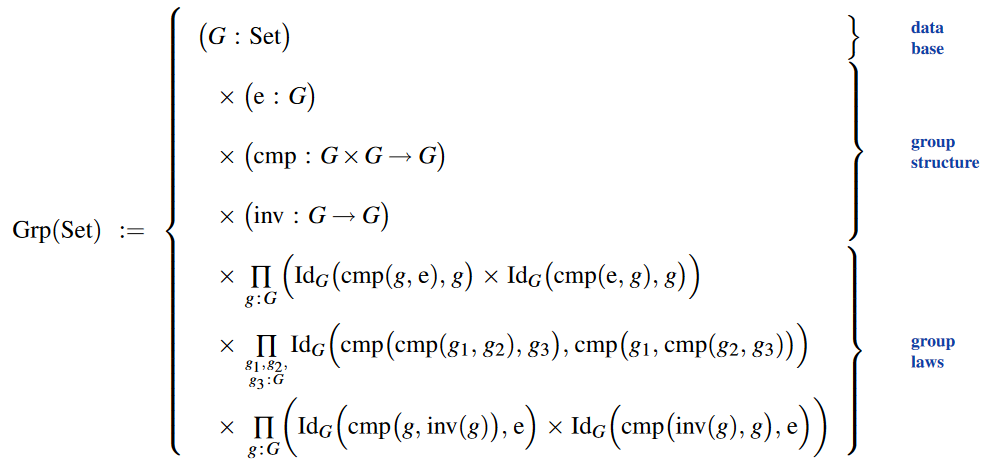# nLab group object

Contents

### Context

#### Categorical algebra

internalization and categorical algebra

universal algebra

categorical semantics

group theory

# Contents

A group object in a category $C$ is a group internal to $C$.

## Definition

### In terms of internal group objects

A group object or internal group in a category $C$ with binary products and a terminal object $*$ is an object $G$ in $C$ and arrows

$1:* \to G$

(the unit map)

$(-)^{-1}:G\to G$

(the inverse map) and

$m:G\times G \to G$

(the multiplication map), such that the following diagrams commute:

$\array{ G\times G\times G & \stackrel{id\times m}{\longrightarrow} & G\times G \\ {}^{ \mathllap{ m\times id } } \big\downarrow && \downarrow m \\ G\times G & \stackrel{m}{\longrightarrow} & G }$

(expressing the fact multiplication is associative),

$\array{ G & \stackrel{(1,id)}{\longrightarrow} & G\times G \\ {}^{\mathllap{(\id,1)}} \big\downarrow &\underset{\id}{\searrow}& \big\downarrow m \\ G\times G & \stackrel{m}{\longrightarrow} &G }$

(telling us that the unit map picks out an element that is a left and right identity), and

$\array{ G & \overset{ ((-)^{-1},id) } {\longrightarrow} & G\times G \\ {}^{ \mathllap{ (id,(-)^{-1}) } } \big\downarrow & \underset{1}{\searrow}& \downarrow m \\ G\times G & \stackrel{m}{\longrightarrow} & G }$

(telling us that the inverse map really does take an inverse).

As a slight abuse of notation we have reused $1$ here to denote $1: G \to G$, defined as the composite $G \to * \stackrel{1}{\to} G$. Also, the associativity law technically factors through the isomorphisms between $(G\times G)\times G$ and $G\times (G\times G)$. The pairing $(f,g)$ denotes $(f\times g)\circ\Delta$ where $\Delta$ is a diagonal morphism.

Even if $C$ doesn't have all binary products, as long as products with $G$ (and the terminal object $*$) exist, then one can still speak of a group object $G$ in $C$.

### In terms of presheaves of groups

###### Proposition

Given a cartesian monoidal category $C$, the category of internal groups in $C$ is equivalent to the full subcategory of the category of presheaves of groups $Grp^{C^{op}}$ on $C$, spanned by those presheaves whose underlying set part in $Set^{C^{op}}$ is representable.

This is a special case of the general theory of structures in presheaf toposes.

In other words, the forgetful functor from $Grp^{C^{op}}$ to $Set^{C^{op}}$ (obtained by composing with the forgetful functor Grp $\to$ Set) creates representable group objects from representable objects.

An object $G$ in $C$ with an internal group structure is a diagram

$\array{ && Grp \\ & {}^{(G,\cdot)}\nearrow & \downarrow \\ C^{op} &\stackrel{Y(G)}{\to}& Set } \,.$

This equips each object $S \in C$ with an ordinary group $(G(S), \cdot)$ structure, so in particular a product operation

$\cdot_S : G(S) \times G(S) \to G(S) \,.$

Moreover, since morphisms in $Grp$ are group homomorphisms, it follows that for every morphism $f : S \to T$ we get a commuting diagram

$\array{ G(S) \times G(S) &\stackrel{\cdot_S}{\to}& G(S) \\ \uparrow^{G(f)\times G(f)} && \uparrow^{G(f)} \\ G(T) \times G(T) &\stackrel{\cdot_T}{\to}& G(T) }$

Taken together this means that there is a morphism

$Y(G \times G) \to Y(G)$

of representable presheaves. By the Yoneda lemma, this uniquely comes from a morphism $\cdot : G \times G \to G$, which is the product of the group structure on the object $G$ that we are after.

etc.

### As data structure

In the language of dependent type theory (using the notation for dependent pair types here) the type of group data structures is:## Theory

The basic results of elementary group theory apply to group objects in any category with finite products. (Arguably, it is precisely the elementary results that apply in any such category.)

The theory of group objects is an example of a Lawvere theory.

The general definition of internal groups seems to have first been formulated in:

following the general principle of internalization formulated in:

reviewed in:

On internalization, H-spaces, monoid objects, group objects in algebraic topology/homotopy theory and introducing the Eckmann-Hilton argument:

With emphasis of the role of the Yoneda lemma:

Review:

In the broader context of internalization via sketches:

With focus on internalization in sheaf toposes: